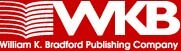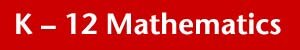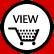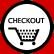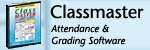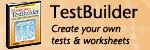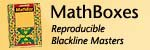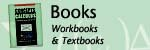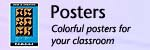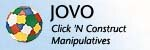Autograph - Graphing Software
leads the way in the use of dependent, selectable mathematical objects to help students come to grips with the basic principles of probability and statistics, and of coordinate geometry in both 2D and 3D.

computer gradebook that calculates, records, reports and forecasts student and class performance, and includes a comprehensive attendance component and seating chart.

MathType 5 - Equation Editor
Using MathType in conjunction with word processing, presentation programs, and HTML authoring software, you can easily create tests, worksheets, and web pages.

Mathematics TestBuilder - Teacher Tool
a powerful authoring tool that combines word processing, database, and layout capabilities for test creation.

Calculus Software
an extensive line of mathematics software, for classroom or home use.

High School Software
an extensive line of mathematics software, for classroom or home use.

Middle School Software
an extensive line of mathematics software, for classroom or home use.

Elementary Software
an extensive line of mathematics software, for classroom or home use. Cocepts of Calculus: Exploring AP Calculus (AB) with the TI-89
written to help students gain an understanding of the most important concepts in Calculus AB and to introduce students to the power of the TI-89 in exploring these concepts graphically, numerically, and analytically.

Cocepts of Calculus: Exploring AP Calculus (BC) with the TI-89
a collection of additional lessons covering Euler's Method, Techniques of Integration, Applications of Differential Equations, Parametric, Polar & Vector Equations, and Sequences & Series.

PreCalculus Textbook
written to help students develop an understanding of mathematics and why it is taught. It bridges the gap between trigonometry and calculus and is ideal for any high school or college student preparing for calculus

Blackline Masters (MathBoxes)
Each MathBox is a fully-developed, ready-to-use supplement for your math curriculum that includes suggestions for integrating the material into your classroom, copymasters for effortless implementation, and answer keys where applicable.

Math Posters

Math Posters